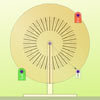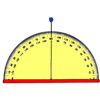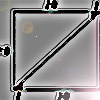# Search by Topic

#### Resources tagged with Sine, cosine, tangent similar to At a Glance:

Filter by: Content type:
Age range:
Challenge level:

### There are 36 results

Broad Topics > Pythagoras and Trigonometry > Sine, cosine, tangent### At a Glance

##### Age 14 to 16 Challenge Level:

The area of a regular pentagon looks about twice as a big as the pentangle star drawn within it. Is it?### Six Discs

##### Age 14 to 16 Challenge Level:

Six circular discs are packed in different-shaped boxes so that the discs touch their neighbours and the sides of the box. Can you put the boxes in order according to the areas of their bases?### Squ-areas

##### Age 14 to 16 Challenge Level:

Three squares are drawn on the sides of a triangle ABC. Their areas are respectively 18 000, 20 000 and 26 000 square centimetres. If the outer vertices of the squares are joined, three more. . . .### Inscribed in a Circle

##### Age 14 to 16 Challenge Level:

The area of a square inscribed in a circle with a unit radius is, satisfyingly, 2. What is the area of a regular hexagon inscribed in a circle with a unit radius?### Dodecawhat

##### Age 14 to 16 Challenge Level:

Follow instructions to fold sheets of A4 paper into pentagons and assemble them to form a dodecahedron. Calculate the error in the angle of the not perfectly regular pentagons you make.### Where Is the Dot?

##### Age 14 to 16 Challenge Level:

A dot starts at the point (1,0) and turns anticlockwise. Can you estimate the height of the dot after it has turned through 45 degrees? Can you calculate its height?##### Age 14 to 16 Challenge Level:

The sides of a triangle are 25, 39 and 40 units of length. Find the diameter of the circumscribed circle.### Circle Scaling

##### Age 14 to 16 Challenge Level:

Describe how to construct three circles which have areas in the ratio 1:2:3.### Moving Squares

##### Age 14 to 16 Challenge Level:

How can you represent the curvature of a cylinder on a flat piece of paper?### Doesn't Add Up

##### Age 14 to 16 Challenge Level:

In this problem we are faced with an apparently easy area problem, but it has gone horribly wrong! What happened?### From All Corners

##### Age 14 to 16 Challenge Level:

Straight lines are drawn from each corner of a square to the mid points of the opposite sides. Express the area of the octagon that is formed at the centre as a fraction of the area of the square.### Raising the Roof

##### Age 14 to 16 Challenge Level:

How far should the roof overhang to shade windows from the mid-day sun?### Figure of Eight

##### Age 14 to 16 Challenge Level:

On a nine-point pegboard a band is stretched over 4 pegs in a "figure of 8" arrangement. How many different "figure of 8" arrangements can be made ?### Muggles, Logo and Gradients

##### Age 11 to 18

Logo helps us to understand gradients of lines and why Muggles Magic is not magic but mathematics. See the problem Muggles magic.### Swings and Roundabouts

##### Age 14 to 16 Challenge Level:

If you were to set the X weight to 2 what do you think the angle might be?### History of Trigonometry - Part 2

##### Age 11 to 18

The second of three articles on the History of Trigonometry.### The History of Trigonometry- Part 1

##### Age 11 to 18

The first of three articles on the History of Trigonometry. This takes us from the Egyptians to early work on trigonometry in China.### History of Trigonometry - Part 3

##### Age 11 to 18

The third of three articles on the History of Trigonometry.### Making Maths: Clinometer

##### Age 11 to 14 Challenge Level:

Make a clinometer and use it to help you estimate the heights of tall objects.### Sine and Cosine for Connected Angles

##### Age 14 to 16 Challenge Level:

The length AM can be calculated using trigonometry in two different ways. Create this pair of equivalent calculations for different peg boards, notice a general result, and account for it.### Circle Box

##### Age 14 to 16 Challenge Level:

It is obvious that we can fit four circles of diameter 1 unit in a square of side 2 without overlapping. What is the smallest square into which we can fit 3 circles of diameter 1 unit?### Orbiting Billiard Balls

##### Age 14 to 16 Challenge Level:

What angle is needed for a ball to do a circuit of the billiard table and then pass through its original position?### 8 Methods for Three by One

##### Age 14 to 18 Challenge Level:

This problem in geometry has been solved in no less than EIGHT ways by a pair of students. How would you solve it? How many of their solutions can you follow? How are they the same or different?. . . .### A Scale for the Solar System

##### Age 14 to 16 Challenge Level:

The Earth is further from the Sun than Venus, but how much further? Twice as far? Ten times?### Eight Ratios

##### Age 14 to 16 Challenge Level:

Two perpendicular lines lie across each other and the end points are joined to form a quadrilateral. Eight ratios are defined, three are given but five need to be found.### Farhan's Poor Square

##### Age 14 to 16 Challenge Level:

From the measurements and the clue given find the area of the square that is not covered by the triangle and the circle.### Coke Machine

##### Age 14 to 16 Challenge Level:

The coke machine in college takes 50 pence pieces. It also takes a certain foreign coin of traditional design...### Lying and Cheating

##### Age 11 to 14 Challenge Level:

Follow the instructions and you can take a rectangle, cut it into 4 pieces, discard two small triangles, put together the remaining two pieces and end up with a rectangle the same size. Try it!### Trigonometric Protractor

##### Age 14 to 16 Challenge Level:

An environment that simulates a protractor carrying a right- angled triangle of unit hypotenuse.### Sine and Cosine

##### Age 14 to 16 Challenge Level:

The sine of an angle is equal to the cosine of its complement. Can you explain why and does this rule extend beyond angles of 90 degrees?### Far Horizon

##### Age 14 to 16 Challenge Level:

An observer is on top of a lighthouse. How far from the foot of the lighthouse is the horizon that the observer can see?### Round and Round a Circle

##### Age 14 to 16 Challenge Level:

Can you explain what is happening and account for the values being displayed?### Round and Round

##### Age 14 to 16 Challenge Level:

Prove that the shaded area of the semicircle is equal to the area of the inner circle.### Screen Shot

##### Age 14 to 16 Challenge Level:

A moveable screen slides along a mirrored corridor towards a centrally placed light source. A ray of light from that source is directed towards a wall of the corridor, which it strikes at 45 degrees. . . .